# How to list all formulas in Excel?

Excel provides various formulas and functions to analyze and examine numerical data. These formulas and functions are mostly aimed at numerical data. An Excel worksheet contains data that is either manually entered or calculated using formulae, but formulas are not visible in the worksheet. We aim to list all the formulas used in an Excel worksheet. In this article, we will describe different ways to list all the formulas present in the given worksheet in Excel. The two major examples can be used to list all the formulae in an Excel worksheet. The Show Formulas option utilize in example 1 and the FORMULATEXT() function is specified in example 2 to obtain the same result.

Let's explore the articles with a few examples.

## Example 1: Using the Show Formulas option.

Let us see the steps involved in using the Show Formulas option.

### Step 1

Click on the tab named Formulas as highlighted in the below image.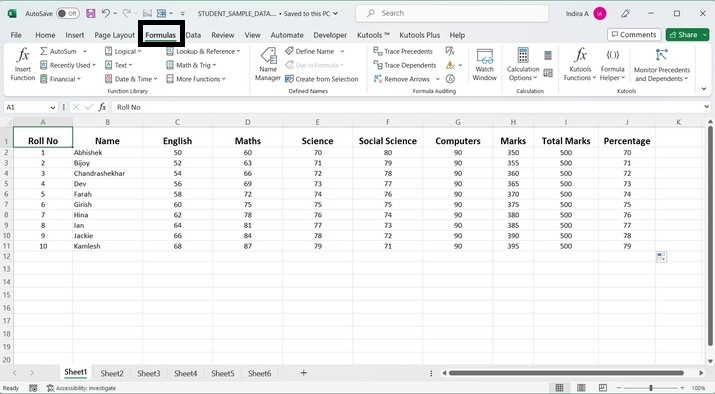### Step 2

Choose the Show Formulas option presented in the “Formulas” tab as shown.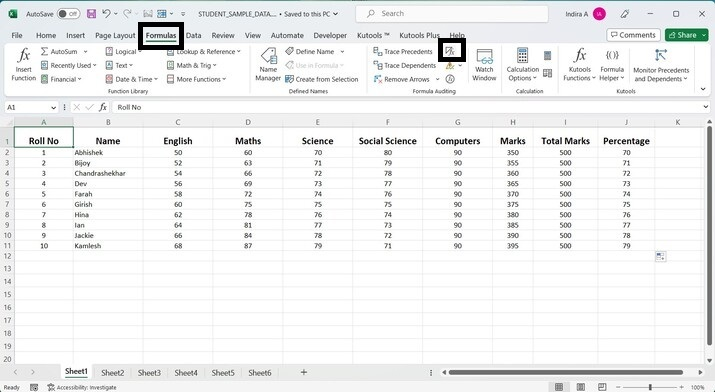### Step 3

Towards the right of the worksheet, two additional columns will be inserted showing the formula for Marks and Percentage columns for each cell.

## Output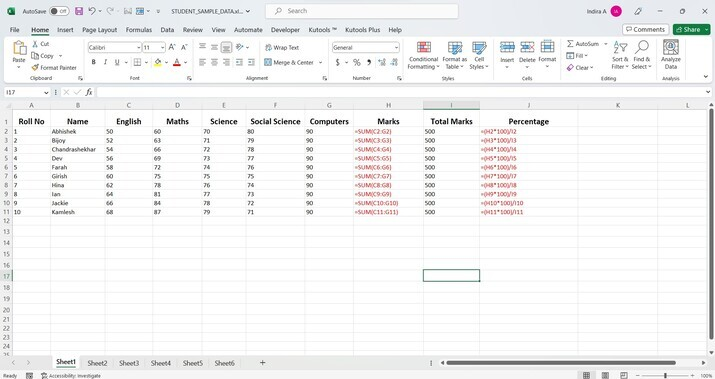As seen from the output image, the formulae used in all the cells of the Marks and Percentage columns have been displayed. They have been highlighted in red color to mark their presence.

## Example 2: Using FORMULATEXT function

Let us look at how we can use FORMULATEXT function to list all the formulas used in the given Excel worksheet.

### Step 1

In the first blank column after the Percentage column, enter the given formula. In this case, the first blank column is column K which is present after the Percentage column.

The formula is as follows.

=FORMULATEXT(H2)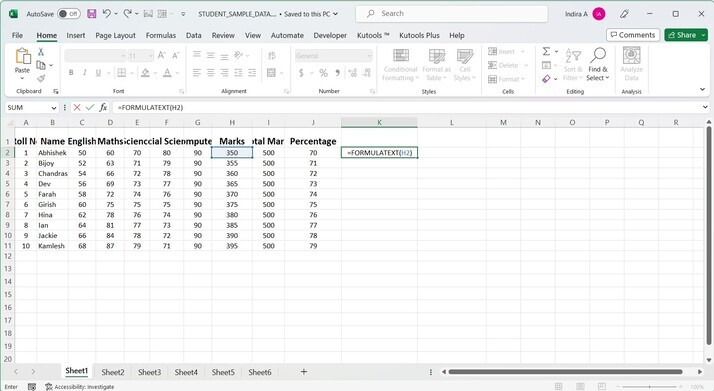### Step 2

After entering the above formula, press Enter key from the keyboard. The formula for calculating Marks for first student will appear in that cell, that is in cell K2.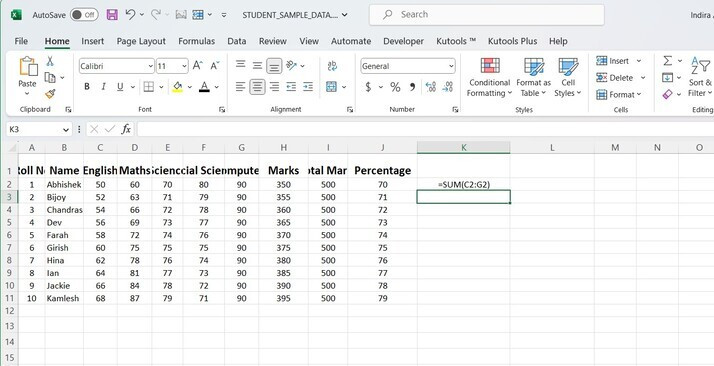### Step 3

Now, use the Fill option to copy this formula in the succeeding cells of column K to show the formulas for the Marks column.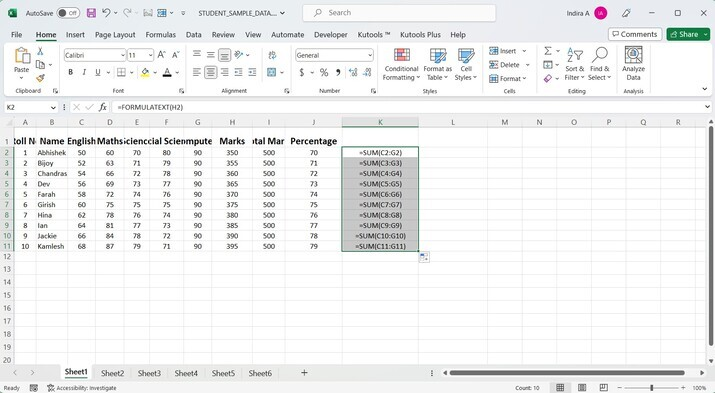As seen from the output image, column K displays all the formulas used to calculate total marks from column H.

### Step 4

Similarly, in column L, in the second cell, that is cell L2, enter the formula given below.

The formula is as follows.

= FORMULATEXT(J2)

###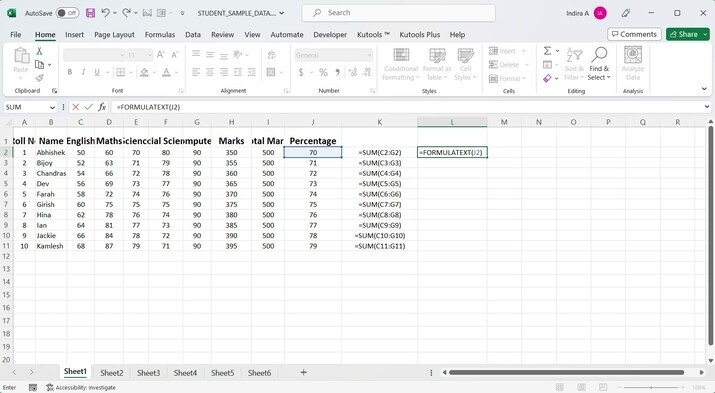Step 5

After entering the formula, press Enter key from the keyboard. This will display the formula used to calculate the percentage of cell J2.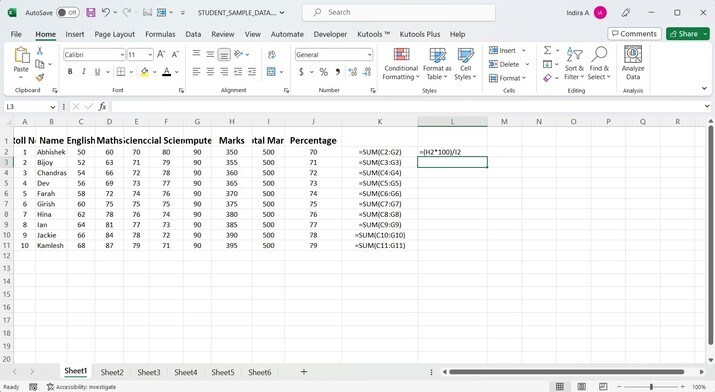### Step 6

Use the Fill option to copy this formula in the succeeding cells of column L to display the formulas for the Percentage column.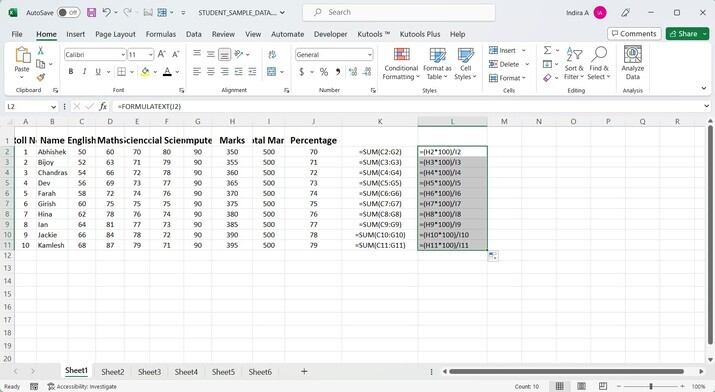As seen from the output image, column L displays all the formulas used to calculate percentages from column J.

## Conclusion

In this article, we described two examples to list all the formulae used in the given Excel worksheet. Also, the user should be able to use formulas in Excel both in the formula bar and in an individual cell. In addition to the above, the user should be familiar with the Fill option in Excel, that is, how to drag and fill the other cells based on the formula entered in the topmost cell.

Updated on: 26-Jul-2023

48 Views